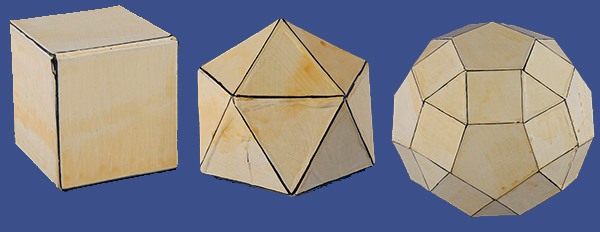# Geometric Models-Regular-Faced Convex PolyhedraFrom ancient times, mathematicians have been intrigued by polyhedra, closed surfaces with polygons as sides. They have been especially interested in those in which the polygons are regular – the sides have the same length and the angles are equal.

In 1970 Martin Berman (1938-1984), a physicist at the Pittsburgh Energy Technology Center, constructed a set of models of regular-faced convex polyhedra. A polyhedron is said to be uniform if its faces are regular and its vertices are all alike (so that it has the same arrangement of polygons at each vertex). A polyhedron (uniform or not) is convex if a line segment joining any two of its points lies entirely on or inside it. Some of these had been studied by mathematicians from ancient times, while others were only enumerated in 1966 by the American mathematician Norman W. Johnson.

Berman made paper models of the Platonic solids, the Archimedean solids, , a prism with a triangular base, and an antiprism with a square base, and the Johnson solids for a total of 112 models. He published photographs of these and diagrams for making them in 1971, identifying the models with the same names as those used by Johnson. Berman gave the models to the Smithsonian in 1978.